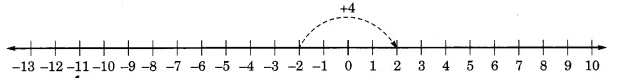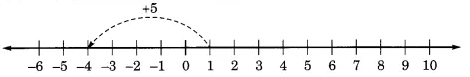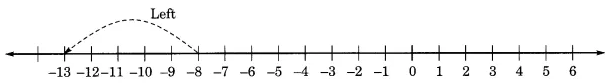Q

# Draw a number line and answer the following: (a) Which number will we reach if we move 4 numbers to the right of - 2.

10.  Draw a number line and answer the following :

(a) Which number will we reach if we move   numbers to the right of
(b) Which number will we reach if we move  numbers to the left of .
(c) If we are at  on the number line, in which direction should we move to reach ?
(d) If we are at on the number line, in which direction should we move to reach

Views

(a)  if we move   numbers to the right of  we get 2.(b)  if we move  numbers to the left of  we get -4(c)  If we are at  on the number line, we should move 5 number to the  left side on the number line.(d)  If we are at on the number line, then we should move 5 number right to the  on the number line.

Exams
Articles
Questions